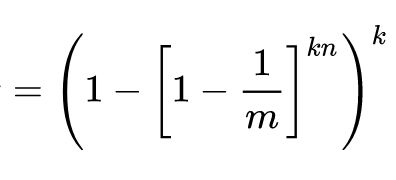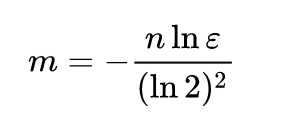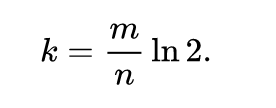# Bloom-Filter

A Bloom filter is a space-efficient probabilistic data structure, conceived by Burton Howard Bloom in 1970, that is used to test whether an element is a member of a set.

An empty Bloom filter is a bit array of m bits, all set to 0. There must also be k different hash functions defined, each of which maps or hashes some set element to one of the m array positions, generating a uniform random distribution.

``````m -> size of bit array
k -> number of hash function
n -> estimated number of element to add in bloom filter
``````

False positive matches are possible, but false negatives are not – in other words, a query returns either "possibly in set" or "definitely not in set".

## Supported Operations

``````  insert(x) : To insert an element in the Bloom Filter.
lookup(x) : to check whether an element is already present
in Bloom Filter with a false positive probability.
``````

NOTE: Delete operation can be supported by using Counting Bloom filter.

## Install

``````go get github.com/akgarhwal/bloomfilter
``````

## Usage

``````// create a new bloom filter
// with 100 elements to be inserted and
// false positive probability of 0.1
bf := bloomfilter.NewBloomFilter(100, 0.1)
bf.Insert([]byte("sachin"))
bf.Insert([]byte("rohit"))
bf.Insert([]byte("virat"))
bf.Insert([]byte("msdhoni"))

// new user name to check

} else {
}
``````

## Probability of False Positive

If m is size of bit array and n is number of elements to be inserted and k is number of hash functions, then the probability of false positive ε can be calculated as:## Size of Bit Array:

If expected number of elements n is known and desired false positive probability is ε then the size of bit array:## Optimal number of hash functions

The number of hash functions k must be a positive integer. If m is size of bit array and n is number of elements to be inserted, then k can be calculated as: